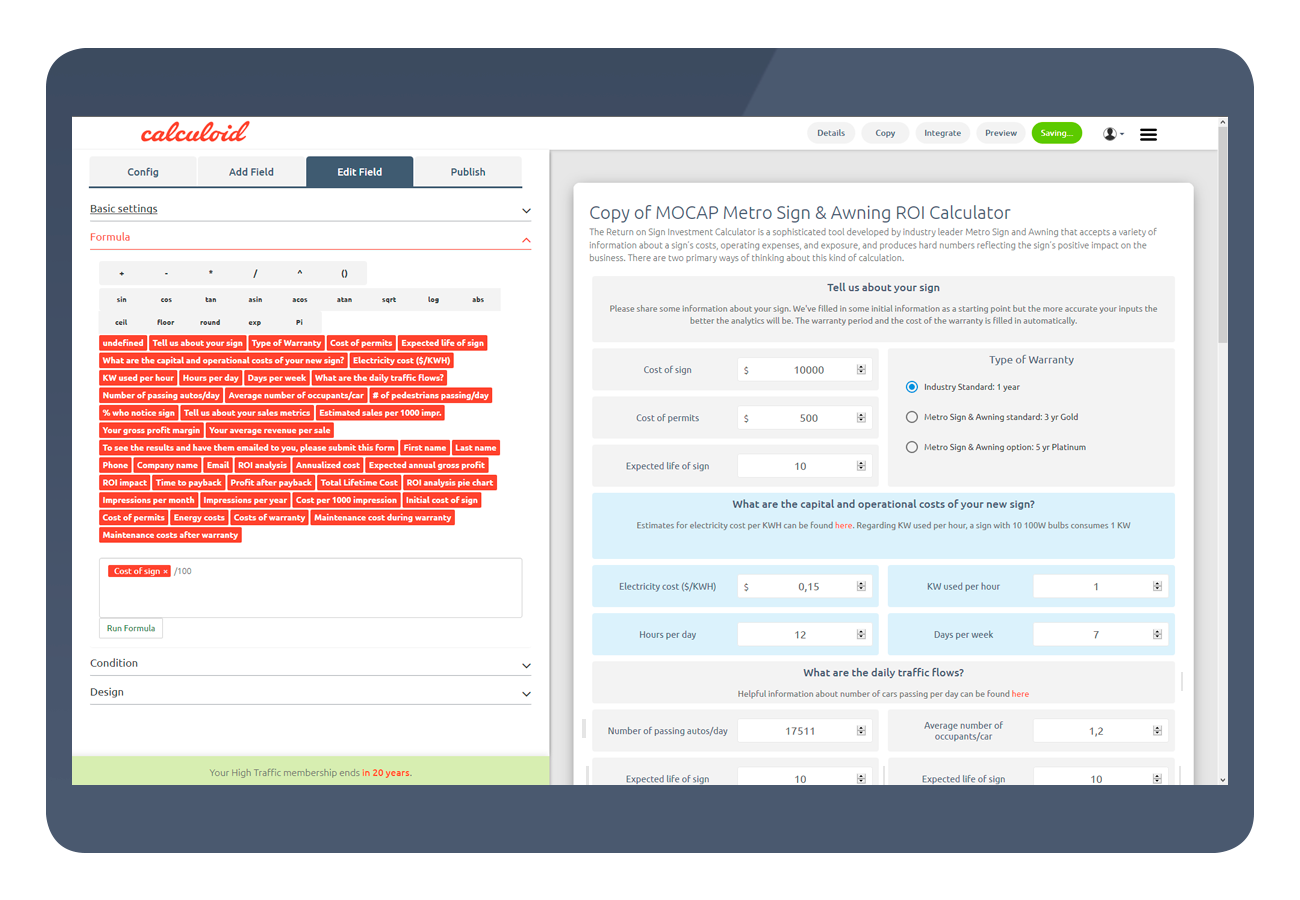Content

In addition to the basic arithmetic calculations, Calculoid allows you to use popular Excel formulas, such as IF, sin, cos, log and others. From basic 1+2 to complex volume discounting, Calculoid has endless capabilities.

Excel-like formulas are used to perform calculations on the values entered to obtain the desired result. There are numerical/mathematical, statistical and logical formulas that make easy work of complex calculations in Calculoid.

Calculoid formula field applies similar logic as Google Spreadsheet and MS Excel, you create the formula by adding fields identifiers into the calculation. In Calculoid you can select fields by their name and add them to the formula with just a click.